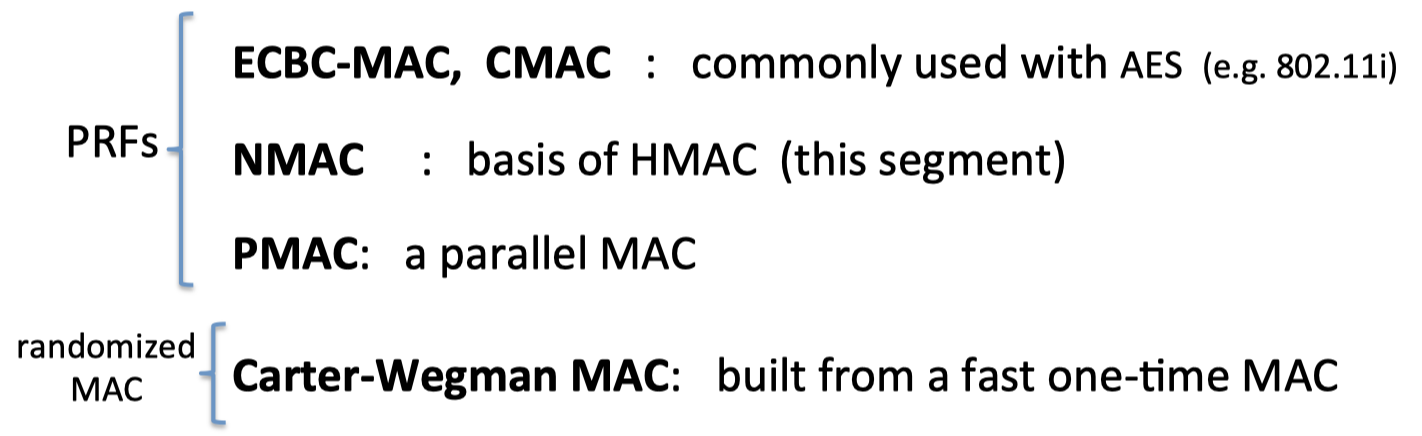# 「Cryptography-MIT6875」: Lecture 7

In this series, I will learn MIT 6.875, Foundations of Cryptography, lectured by Vinod Vaikuntanathan.
Any corrections and advice are welcome. ^ - ^

It is indeed possible for $F(x)$ to leak a lot of information about $x$ even if $F$ is one-way.

The hardcore predicate $B(x)$ represent the specific piece of information about $x$ which is hard to compute given $F(x)$.

Topics Covered:

• Definition of one-way functions (OWF)
• Definition of hardcore bit/predicate (HCB)
• One-way permutations → PRG.
(In fact, one-way functions → PRG, but that’s a much harder theorem.)
• Goldreich-Levin Theorem: every OWF has a HCB.
(Proof for an important special case.)

# 「Cryptography-MIT6875」: Lecture 6 - Number Theory

In this series, I will learn MIT 6.875, Foundations of Cryptography, lectured by Vinod Vaikuntanathan.
Any corrections and advice are welcome. ^ - ^
The motif of this blog is Number Theory, including the second half of Lecture 5 and the Lecture 6.

It’s an excellent opportunity to learn Number Theory in manner of English.

An important point in this blog is that we focus more on the statements, which is useful in later lectures, rather than the proof.

About 70% of the content in this blog is originally and literally from the lecture notes. I just organize and refine it according to the logic of the professor’s narration since the lecture note is awesome.

The rest is my own understanding and derivation of some theorems. And I will be learning Number Theory and completing the omitted proof.

So this blog will be updated continuously.

Topics covered:

• Groups, Order of a group and the Order of an element, Cyclic Groups.
• The Multiplicative Group $\mathbb{Z}_N^*$ and $\mathbb{Z}_P^*$ for a prime $P$.
• Generators of $\mathbb{Z}_P^*$.
• Primes, Primality Testing.
• The Discrete Logarithm (DLOG) problem and a candidate OWF.
• Diffie-Hellman assumptions: DDH and CDH.

# 「Cryptography-MIT6875」: Lecture 5

In this series, I will learn MIT 6.875, Foundations of Cryptography, lectured by Vinod Vaikuntanathan.
Any corrections and advice are welcome. ^ - ^

Topics covered:

• Applications of PRFs

Note: The second half of Lecture 5, Number Theory, is contained in Lecture 6.

# 「Cryptography-MIT6875」: Lecture 4

In this series, I will learn MIT 6.875, Foundations of Cryptography, lectured by Vinod Vaikuntanathan.
Any corrections and advice are welcome. ^ - ^

Topics:

• Stateless Secret-key Encryption with PRF.
• The Goldreich-Goldwasser-Micali (GGM) PRF construction.

# 「Cryptography-MIT6875」: Lecture 3

In this series, I will learn MIT 6.875, Foundations of Cryptography, lectured by Vinod Vaikuntanathan.
Any corrections and advice are welcome. ^ - ^

Topics:

• The Hybrid Argument.
• An application: PRG length extension.
• The notion of pseudorandom functions: Definition, motivation, discussion and comparison with PRGs.
• PRG implies (stateful) secret-key encryption.
• PRFs imply (stateless) secret-key encryption.

# 「Cryptography-MIT6875」: Lecture 2

In this series, I will learn MIT 6.875, Foundations of Cryptography, lectured by Vinod Vaikuntanathan.
Any corrections and advice are welcome. ^ - ^

Science wins either way.

Topics:

• How to circumvent Shannon’s lower bound: the computational adversary
• Definition of computational security
• The definition of pseudorandom generators(PRG)

# 「Cryptography-MIT6875」: Lecture 1

In this series, I will learn MIT 6.875, Foundations of Cryptography, lectured by Vinod Vaikuntanathan.
Any corrections and advice are welcome. ^ - ^

Everything you’ve ever wanted is on the other side of fear.

Topics:

• Introduction to cryptography
• Secure Communication and Shannon’s definitions of perfect secrecy
• Perfect Indistinguishability definitions.
• Shannon’s lower bound.

# 「Cryptography-Boneh」:Collision Resistance# 「Cryptography-Boneh」:Integrity

• 串行构造：CBC-MAC、NMAC、CMAC
• 并行构造：PMAC、HMAC（下一篇文章）
• 基于one-time MAC: CW MAC